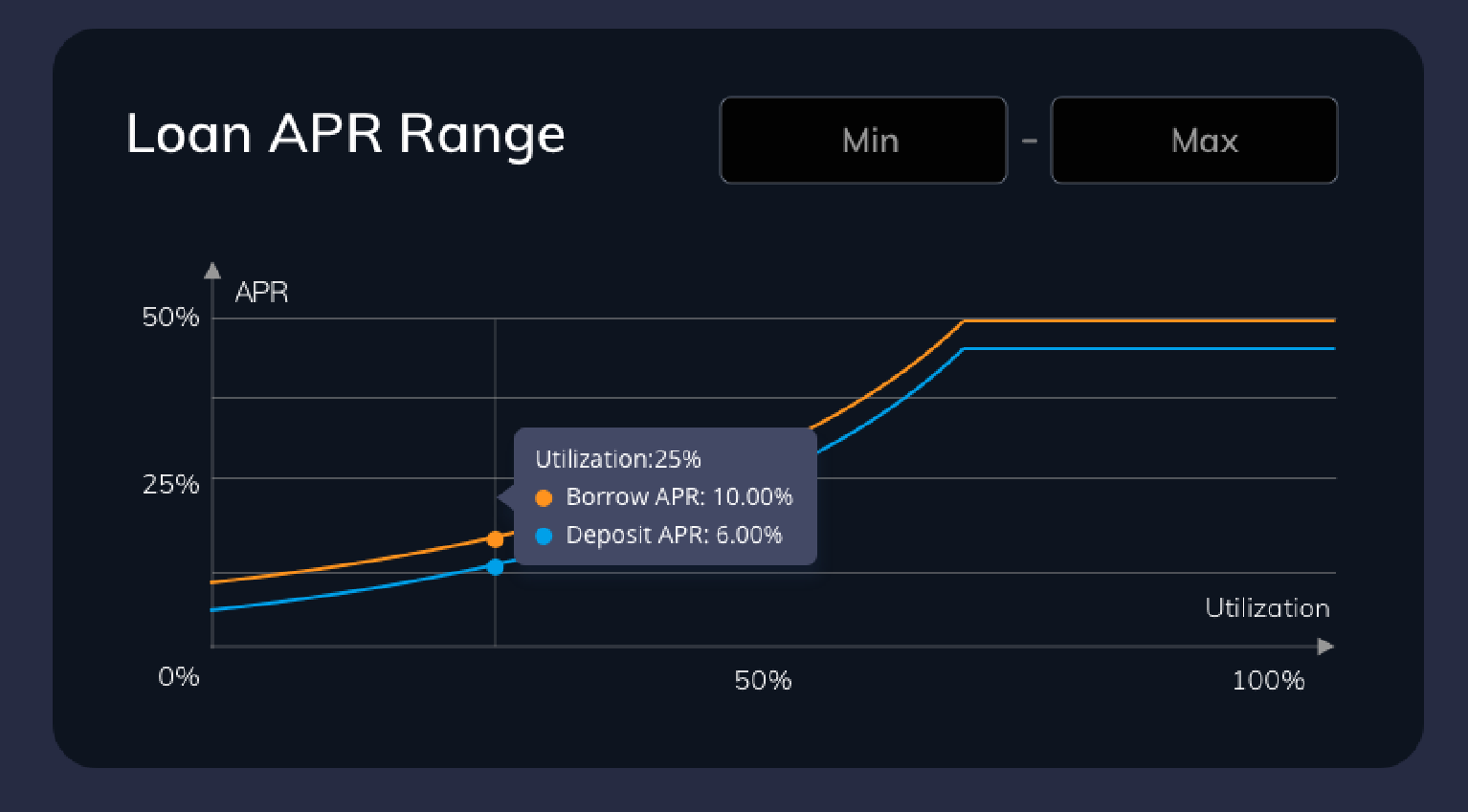# Loan APR Range### What is Loan APR Range?

The loan APR range is for creators to choose the annual percentage return rate range for deposits and loans. The curve is a piecewise function curve. The minimum APR a creator can choose is 3% and the maximum is 300%
• Deposit APR (Annual Percentage Rate) is the annual rate of return earned on a deposit, without taking into account the effect of compounding interest.
• Borrow APR (Annual Percentage Rate) is the annual rate of interest paid on borrow funds, without taking into account the effect of compounding interest.

### How is the APR calculated?

The loan APR range can be adjusted by two parameters: RateCurveConstant and maximum utilization rate.
• Borrow APR = RateCurveConstant÷(1−u), when utilization rate is larger than maximum utilization rate(umax)